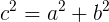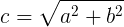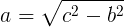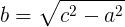# Pythagorean theorem calculator

Hypotenuse (c) calculation:

 Enter leg (a): Enter leg (b): Hypotenuse (c) result:

Leg (a) calculation:

 Enter leg (b): Enter Hypotenuse (c): Leg (a) result:

Leg (b) calculation:

 Enter leg (a): Enter hypotenuse (c): Leg (b) result:

## Pythagorean theorem

For right triangle: the square value the hypotenuse (c) is equal to the sum of the square value of leg (a) and the square value of leg (b):### Hypotenuse (c) calculation### Leg (a) calculation### Leg (b) calculation## Features of Pythagorean theorem Calculator

Our Pythagorean theorem Calculator allows the users to Calculate Pythagorean theorem. Some of the prominent features of this utility are explained below.

#### No Registration

You don’t need to go through any registration process to use the Pythagorean theorem Calculator. Using this utility, users to Calculate Pythagorean theorem as many times as you want for free.

#### Fast conversion

This Pythagorean theorem Calculator offers users the fastest Calculate. Once the user enters the  Pythagorean theorem values ​​in the input field and clicks the Calculate button, the utility will start the conversion process and return the results immediately.

#### Saves Time and Effort

The manual procedure of Calculator Pythagorean theorem isn’t an easy task. You must spend a lot of time and effort to complete this task. The Pythagorean theorem Calculator allows you to complete the same task immediately. You will not be asked to follow manual procedures, as its automated algorithms will do the work for you.

##### Accuracy

Despite investing time and effort in manual Calculation, you might not be able to get your hands on accurate results. Not everyone is good at solving math problems, even if you think you're a pro, there's still a good chance you'll get in accurate results. This situation can be smartly handled with the help of a Pythagorean theorem Calculator. You will be provided with 100% accurate results by this online tool.

##### Compatibility

The online Pythagorean theorem converter perfectly works on all operating systems. Whether you have a Mac, iOS, Android, Windows, or Linux device, you can easily use this online Tool without facing any hassle.

###### 100% Free

You don't need to go through any registration process to use this Pythagorean theorem Calculator. You can use this utility for free and do unlimited Pythagorean theorem Calculate without any limitations.